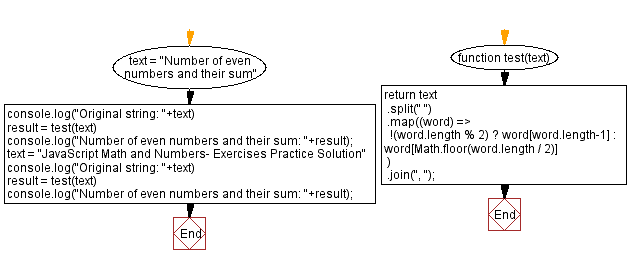# JavaScript: Last and middle character from a string

## JavaScript Math: Exercise-95 with Solution

Write a JavaScript program that takes text and returns the last character of each word if it is even in length. It also returns the middle character if it is odd in length.

Test Data:
("Number of even numbers and their sum") -> "r, f, n, b, n, e, u"
("JavaScript Math and Numbers- Exercises Practice Solution") -> "t, h, n, -, c, e, n"

Sample Solution:

HTML Code:

``````<!DOCTYPE html>
<html>
<meta charset="utf-8">
<title>JavaScript program to Last and middle character from a string</title>
<body>

</body>
</html>
```
```

JavaScript Code:

``````function test(text) {
return text
.split(" ")
.map((word) =>
!(word.length % 2) ? word[word.length-1] : word[Math.floor(word.length / 2)]
)
.join(", ");
}
text = "Number of even numbers and their sum"
console.log("Original string: "+text)
result = test(text)
console.log("Number of even numbers and their sum: "+result);
text = "JavaScript Math and Numbers- Exercises Practice Solution"
console.log("Original string: "+text)
result = test(text)
console.log("Number of even numbers and their sum: "+result);
```
```

Sample Output:

```Original string: Number of even numbers and their sum
Number of even numbers and their sum: r, f, n, b, n, e, u
Original string: JavaScript Math and Numbers- Exercises Practice Solution
Number of even numbers and their sum: t, h, n, -, c, e, n
```

Flowchart:Live Demo:

See the Pen javascript-math-exercise-95 by w3resource (@w3resource) on CodePen.

Improve this sample solution and post your code through Disqus

What is the difficulty level of this exercise?

Test your Programming skills with w3resource's quiz.

﻿